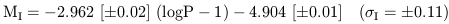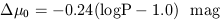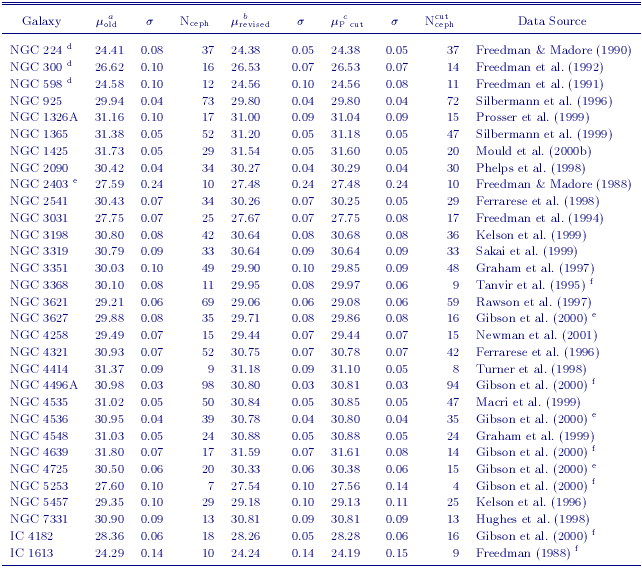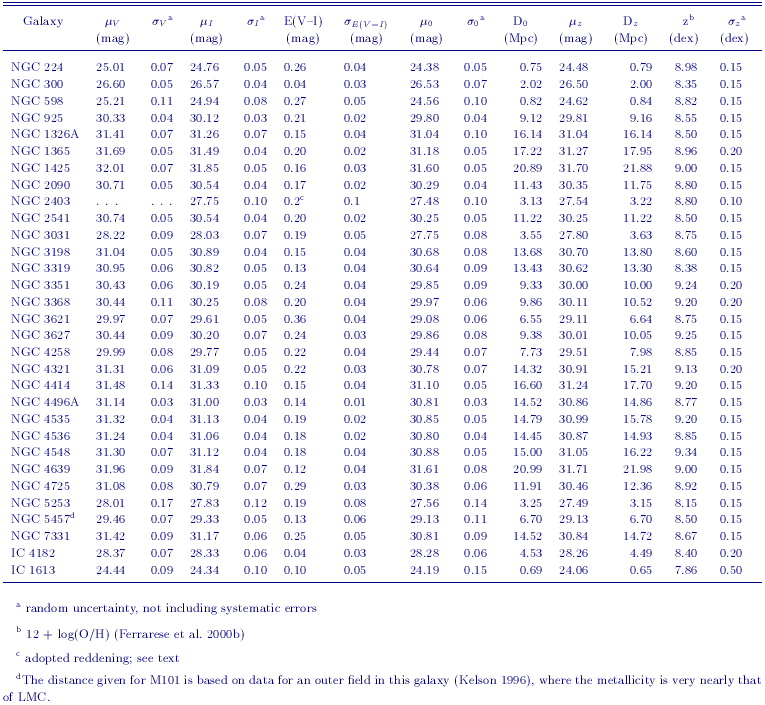### 3. THE CEPHEID DISTANCE SCALE

The Cepheid period-luminosity relation remains the most important of the primary distance indicators for nearby galaxies. The strengths and weaknesses of Cepheids have been reviewed extensively (e.g., Feast & Walker 1987; Madore & Freedman 1991; Jacoby et al. 1992; Freedman & Madore 1996; Tanvir 1999). However, since the Cepheid distance scale lies at the heart of the H0 Key Project, we summarize both its advantages and disadvantages briefly here again.

The strengths of Cepheids are, of course, many: they are amongst the brightest stellar indicators, and they are relatively young stars, found in abundance in spiral galaxies. Thus, many independent objects can be observed in a single galaxy. Their large amplitudes and characteristic (saw-tooth) light curve shapes facilitate their discovery and identification; and they have long lifetimes and hence, can be reobserved at other times, and other wavelengths (unlike supernovae, for example). The Cepheid period-luminosity relation has a small scatter (e.g., in the I-band, the dispersion amounts to only ~ ± 0.1 mag: Udalski et al. 1999). Moreover, Cepheids have been studied and theoretically modelled extensively; the reason for their variability is well-understood to be a consequence of pulsation of the atmosphere, resulting from a thermodynamic, valve-like driving mechanism as (primarily) helium is cycled from a singly to doubly ionized state, and the opacity increases with compression.

There are also difficulties associated with measuring Cepheid distances. First, since Cepheids are young stars, they are found in regions where there is dust scattering, absorption and reddening. Corrections must be made for extinction, requiring assumptions about the universal behavior of Cepheids at different wavelengths, and about the universality of the Galactic extinction law. Extinction is systematic, and its effects must either be removed by multicolor data, or minimized by observing at long wavelengths, or both. Second, the dependence of the PL relation on chemical composition (metallicity) has been very difficult to quantify. Third, an accurate geometric calibration of the PL relation, at any given metallicity, has not yet been established. Fourth, as the distance of the galaxy increases (and the resolution decreases), finding and measuring individual Cepheids becomes increasingly difficult due to crowding effects. Finally, the reach of Cepheids is currently (with HST) confined to spiral galaxies with distances less than about 30 Mpc. Hence, Cepheids alone cannot be observed at sufficient distances to determine H0 directly, and an accurate determination of H0 requires an extension to other methods.

The application of the PL relation for the Key Project follows the procedure developed in Freedman (1988), and extended in Freedman, Wilson & Madore (1991). The Large Magellanic Cloud (LMC) PL relation has been used as fiducial, and a distance modulus of µ0 = 18.50 mag (a distance of 50 kpc), and a mean reddening of E(V-I) = 0.13 (E(B-V) = 0.10) mag (Madore & Freedman 1991) have been adopted. The LMC V- and I- band PL relations are fit by least-squares to the target spiral data to determine apparent distance moduli in each band. A reddening-corrected distance modulus and differential absorption with respect to the LMC are obtained using a ratio of total-to-selective absorption R = AV / (AV - AI) = 2.45 (e.g., Cardelli, Mathis & Clayton 1989). This procedure is equivalent to defining a reddening-free index W = V - R (V-I) (Madore 1982; Freedman 1988; Freedman, Wilson & Madore 1991).

A longstanding uncertainty in the Cepheid distance scale has been the possibility that the zero point of the PL relation is sensitive to chemical composition (Freedman & Madore 1990 and references therein). It is only within the last decade or so, that major observational efforts to address the metallicity issue for Cepheids have been undertaken. Accurately establishing the size of a metallicity effect for Cepheids alone has proven to be very challenging, and the issue has not yet been definitively resolved (see Freedman et al. 2001 and references therein). However, although neither the magnitude of the effect nor its wavelength dependence have yet been firmly established, the observational and theoretical evidence for an effect is steadily growing. Published empirical values for the index(see Equation 5 in Section 3.3 below) range from 0 to -1.3 mag/dex (with most values between 0 and -0.4), but these published values have been derived using a variety of different combinations of bandpasses. Since the effects of metallicity are wavelength-dependent, it is critical that the appropriate correction for a given dataset be applied.

Some recent theoretical models (e.g., Chiosi, Wood & Capitanio 1993; Sandage, Bell & Tripicco 1999; Alibert et al. 1999; Bono et al. 1999, 2000) suggest that at the VI bandpasses of the H0 Key Project, the effect of metallicity is small,VI ~ -0.1 mag/dex. Unfortunately, the sign of the effect is still uncertain. For example, Caputo, Marconi & Musella (2000) find a slope of 0.27 mag/dex, with the opposite sign. Thus, for the present, calibrating the metallicity effect based on models alone is not feasible.

A differential, empirical test for the effects of metallicity on the Cepheid distance scale was first carried out by Freedman & Madore (1990) for the nearby galaxy M31. As part of the Key Project, we carried out a second differential test comparing two fields in the face-on galaxy, M101 (Kennicutt et al. 1998). These two studies are consistent with there being a shallow metallicity dependence, but the statistical significance of each test is individually low. As a follow-on to the optical study, H-band NICMOS observations have been obtained for the two fields previously observed in the optical in M101 (Macri et al. 2001). A comparison of the VIH photometry for the inner and outer field is consistent with a metallicity sensitivity of the PL relations, but artificial star tests in the inner field indicate that crowding is significant, and precludes an accurate determination of the magnitude of the effect. Other recent studies (e.g., Sasselov et al. 1997; Kochanek 1997) conclude that a metallicity effect is extant, and all of the empirical studies agree on the sign, if not the magnitude of the effect. Considering all of the evidence presently available and the (still considerable) uncertainties, we therefore adoptVI = -0.2 ± 0.2 mag/dex, approximately the mid-range of current empirical values, and correct our Cepheid distances accordingly.

For earlier papers in this series, we adopted the slopes and zero points for the LMC V- and I- PL relations from Madore & Freedman (1991), based on 32 Cepheids. These PL relations are consistent with those published by Feast & Walker (1987). However, the OGLE survey has recently produced a significantly larger sample of ~ 650 LMC Cepheids (Udalski et al. 1999). This sample has extensive phase coverage at BVI magnitudes and covers the period range of 0.4 < log P < 1.5. As part of the Key Project, we also undertook observations of a sample of 105 LMC Cepheids (Sebo et al. 2001), and these PL relations are in very good statistical agreement with those of Udalski et al. adjusting to a common distance to the LMC. For about 60 objects common to both samples, with P > 8 days and having both V and I magnitudes, the offsets are -0.004 ± 0.008 mag in I, and +0.013 ± 0.010 mag in V (Sebo et al. ). The Sebo et al. sample extends to longer periods (~ 40 days), and has 10 Cepheids with periods greater than 30 days, the limit of the Udalski et al. sample. These 10 Cepheids are all well fit by, and all lie within 1-of the period-luminosity slopes defined by the Udalski et al. sample. The Udalski et al. data are clearly the most extensive to date, and we thus adopt their apparent PL relations as fiducial for the reanalysis in this paper.

The Udalski et al. (1999) PL calibration adopts a distance modulus of 18.2 mag, based on a distance determined using the red clump technique, whereas, as discussed above, in this paper, we adopt a true distance modulus to the LMC of 18.50 mag. With this modulus and the reddening-corrected Udalski et al. Cepheid data to define the slopes and errors, our adopted MV and MI PL relations become:(1)(2)

In the absence of a metallicity dependence, and correcting only for reddening, the true distance moduli (µ0) can be calculated from the apparent V and I distance moduli (µV and µI) as follows:(3)(4)

As discussed in more detail in Section 3.4, it is the change in slope of the I-band PL relation that has the most impact on the resulting distances.

Allowing for a correction termµz for a metallicity-dependence of the Cepheid PL relation in terms of the observed HII region abundance of oxygen relative to hydrogen (see Section 8.3), the true distance modulus becomes:(5)

whereµz =VI ([O/H] - [O/H]LMC) is applied to the reddening-corrected (VI) modulus, andVI is measured in mag/dex (where a dex refers to a factor of 10 difference in metallicity).

Over the 6 years that we have been publishing data from the Key Project, our analysis methods, as well as the photometric calibration, have evolved and improved. Hence, the sample of published Key Project distances has not been analyzed completely homogeneously. In this paper, we have redetermined the true moduli to each galaxy used in the Key Project. These distances are calculated with the new calibration described above, and with attention to minimizing bias at the short-period end of the PL relation, as described below and by Freedman et al. 1994b; Kelson et al. 1994; Ferrarese et al. 2000b.

In this analysis we have (1) consistently adopted only the published Cepheid photometry which were reduced using the ALLFRAME stellar photometry reduction package, whose phase points were converted to mean magnitudes using intensity-weighted averages (or their template-fitted equivalents). 18 (2) To compensate for the small (~ 0.01 mag) mean bias in the PL fits (see the discussion in Section 8.4 and Appendix A), we have also applied period cuts to the PL relations, thereby eliminating the shortest-period Cepheids where magnitude incompleteness effects become important. In two cases (NGC 3368 and NGC 300), a single long-period Cepheid was also dropped because of stochastic effects at the bright (sparsely populated) end of the PL relation, which can similarly bias solutions. The mean correction for this magnitude-limited bias is small (+1% in distance), but it is systematic, and correcting for it results in larger distances than are determined without this faint-end debiasing. (3) We have adopted a -0.07 mag correction to the Hill et al. (1998) WFPC2 calibration to be consistent with Stetson (1998) and Dolphin (2000). Finally, (4) we have adopted the published slopes of the Udalski et al. (1999) PL relations.

The adoption of the new Udalski et al. (1999) PL slopes alone has a dramatic, and unanticipated effect on the previously published Cepheid distances based on the Madore & Freedman (1991) calibration. Most importantly, the change is distance dependent. The V and I PL slopes for the Madore & Freedman calibration, based on 32 stars, are -2.76 ± 0.11 and -3.06 ± 0.07, respectively. The new Udalski et al. (1999) values for these same quantities are -2.76 ± 0.03 and -2.96 ± 0.02, (Equations 1 and 2). Although the V-band slopes agree identically, and the I-band slopes differ by only 0.1, the impact on the derived reddenings, and therefore distances, is significant. The new calibration predicts higher reddenings, and therefore smaller distances. In addition, because of the difference in (V-I) slope, the new relation predicts systematically larger reddenings for Cepheids of increasing period. As a result, the differences in distance between the previous and the new calibration will be largest for galaxies at greater distances, where the mean period of the samples is larger (since a greater fraction of shorter-period Cepheids will fall below the detection threshold in the most distant targets).

Expressing the divergence of the two calibrations as a correction to the true modulus (in the sense of Udalski et al. (1999) minus Madore & Freedman (1991)):(6)

The two calibrations agree at around 10 days in period. At 20 days the correction amounts to less than a 4% decrease in distance. At 30 days, this difference is 6%, and it rises to 9.5% (or -0.19 mag in distance modulus) at 60 days.

In Table 3, this new calibration is applied to all KP galaxies and other Cepheid distances from HST observations. Corrections for metallicity are applied in Table 4. In addition, we present revised VI moduli for M33, M31, IC 1613, NGC 300, and I-band for NGC 2403. These galaxies were previously observed from the ground, and with the exception of IC 1613 (which was observed with NICMOS), have also been used as calibrators for secondary methods for the Key Project. We have not included other dwarf galaxies (like NGC 6822 or WLM) which are not calibrators for the secondary methods adopted in this paper. The fits were done using the same standard procedure described in Section 3.1, and adopting Equation 3. To make it clear where the differences lie compared to previous calibrations, we list in columns 1-4 the galaxies, distance moduli, errors, and number of Cepheids fit, based on the Madore & Freedman (1991) LMC PL relations, and ALLFRAME magnitudes, for an LMC distance modulus of 18.50 mag. In columns 5 and 6, we list distance moduli and errors for fits to the same Cepheid samples adopting the Udalski et al. (1999) PL slopes. In columns 7, 8, and 9, distance moduli, errors, and number of Cepheids fit are given, after imposing period cuts correcting for PL bias as described above. Finally, the references to the sources for the Cepheid photometry is given in column 10. In Table 4, we list the galaxy name, apparent V and I distance moduli and PL-fitting (random) errors, E(V-I), distance moduli on the new calibration for the case where no metallicity correction has been applied (µz = 0), and where a correction ofµz = -0.2 mag/dex is adopted. In addition, we list the distance in Mpc and the metallicities for the Cepheid fields. For ease of comparison, columns 7 of Table 3, and 8 of Table 4, are the same distance moduli values, uncorrected for metallicity.The errors on the Cepheid distances are calculated as follows. The random uncertainties,2random are given by:where N is the number of Cepheids observed in a given galaxy. The error in W,W2 includes the random errors in the photometry minus the correlated scatter along a reddening trajectory (from equation 3). The systematic errors are given by:with corresponding terms due to the uncertainty in the LMC zero point, metallicity, the photometric zero point and aperture corrections. A further discussion of errors can be found in Madore et al. 1999; Ferrarese et al. 2000b.

There are 3 interesting effects of the differential distance-dependent effect in adopting the new Udalski et al. (1999) calibration. First, the absolute magnitudes of the Type Ia supernovae, which previously produced lower values of the Hubble constant in comparison to the other Key Project secondary distance indicators now come into systematically better correspondence (Section 6.1). Second, another apparent divergence in the Cepheid distance scale is also ameliorated by this new calibration; that of the difference between the maser and the Cepheid distance to NGC 4258. As discussed further in Section 8.1.1, adopting the Key Project fitting methodology, ALLFRAME photometry, template-fitted magnitudes, and the new calibration, the Cepheid distance to NGC 4258 comes into better agreement with the maser distance to this galaxy (Herrnstein et al. 1999). And third, the reddening solutions for two galaxies, NGC 300 and IC 4182 previously yielded negative values. The adoption of the new Udalski et al. (1999) slopes results in positive reddening solutions for both these (and now all) galaxies with measured Cepheid distances.

18 For Key Project galaxies, both phase-weighted and intensity-weighted magnitudes were generally calculated for each of the galaxies, and found to be in very good agreement. This is to be expected, since the optimal scheduling results in well-sampled phase coverage. Back.